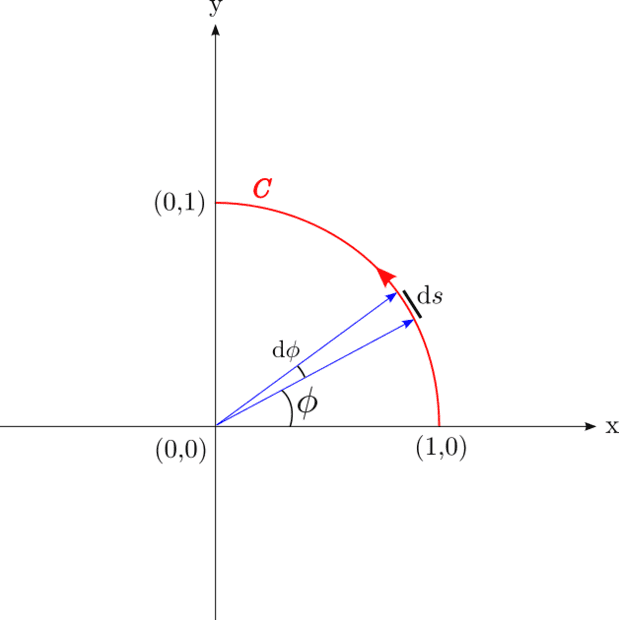• brotherbobby
In summary, the problem requires us to show that the line integral ##I = \int_C x^2y\;ds = \frac{1}{3}##, and the solution is approached in three ways: using (plane) polar coordinates, cartesian coordinates with x as the integral variable, and cartesian coordinates with y as the integral variable. While each approach yields the correct answer, drawing a diagram can be helpful in visualizing the problem. Additionally, it is important to pay attention to the signs when dealing with line integrals.

#### brotherbobby

Homework Statement
Show that ##\pmb{\int_C x^2y\; ds = \frac{1}{3}}## where ##C## is the circular arc in the first quadrant of the unit circle.
Relevant Equations
(1) The line element ##ds^2=dx^2+dy^2\Rightarrow ds = \sqrt{1+\left( \frac{dy}{dx} \right)^2} dx## in cartesian coordinates.
(2) The line element ##ds = a d\phi##, where ##a## is the radius of the circle, in polar coordinates. (Here ##a=1\Rightarrow ds = d\phi##).
(3) The equation of the quadrant is : ##x^2 + y^2 = 1## with ##0\le (x,y) \le 1##Problem : We are required to show that ##I = \int_C x^2y\;ds = \frac{1}{3}##.

Attempt : Before I begin, let me post an image of the problem situation, on the right. I would like to do this problem in three ways, starting with the simplest way - using (plane) polar coordinates.

(1) In (plane) polar coordinates : Remembering that the radius of the (circular) quadrant is 1 (##a=1##), we have the replacements : ##x = \cos \phi\,\, , y = \sin \phi\;\; , ds = d\phi##. The limits of the integral vary from ##\phi = 0\rightarrow \pi/2##, where (I assume) that the quadrant is navigated in an anti- clockwise manner.

Hence the line integral ##I = \int_{0}^{\pi/2} \cos^2 \phi \sin\phi \; d\phi##. Choosing ##z=\cos\phi## makes ##dz = -\sin \phi d\phi## and the limits going from 1 to 0. Our integral becomes ##I = - \int_1^0 z^2 dz = \int_0^1 z^2 dz = \frac{z^3}{3}\Biggr|_0^1 = \boxed{\frac{1}{3}}##, which yields the answer easily.

##\boxed{\text{This is where I run into a small problem}}##

(2) Using cartesian coordinates with x as the integral variable : The line integral ##I = \int_C x^2 y \; ds##. My aim is to convert everything inside the integral sign into the variable ##x##. Clearly, ##y = \sqrt{1-x^2}##, from the equation of the (circular) quadrant. As for ##ds^2=dx^2+dy^2\Rightarrow ds = \sqrt{1+\left( \frac{dy}{dx} \right)^2} dx##. Using ##y=\sqrt{1-x^2}\Rightarrow \frac{dy}{dx} = \frac{-x}{\sqrt{1-x^2}}##. This makes sense because the slope of the ##ds## is negative in the first quadrant (see small black line in picture representing the direction of ##ds##).
Thus ##1+\left (\frac{dy}{dx} \right)^2 = 1+\frac{x^2}{1-x^2} = \frac{1}{1-x^2}##. Thus, we have ##ds = \frac{1}{\sqrt{1-x^2}}dx\Rightarrow yds = dx##.

Putting the information from above, the integral ##I = \int_{x=1}^{x=0} x^2 dx = \boxed{-\frac{1}{3}}##
(which is wrong by the minus ##-## sign}.

A hint or help would be welcome.

##\boxed{\text{Curiously, things work out fine if I use cartesian coordinates treating y as the integral variable}}##

(3) Using cartesian coordinates with y as the integral variable : The line integral ##I = \int_C x^2 y \; ds##. My aim is to convert everything inside the integral sign into the variable ##y##. Clearly, ##x = \sqrt{1-y^2}\Rightarrow x^2 = 1-y^2##, from the equation of the (circular) quadrant. As for ##ds^2=dx^2+dy^2\Rightarrow ds = \sqrt{1+\left( \frac{dx}{dy} \right)^2} dy##. Using ##x=\sqrt{1-y^2}\Rightarrow \frac{dx}{dy} = \frac{-y}{\sqrt{1-y^2}}##. Thus ##\left( 1+\frac{dx}{dy} \right)^2 = 1+\frac{y^2}{1-y^2} = \frac{1}{1-y^2}##. Thus, we have ##ds = \frac{dy}{\sqrt{1-y^2}}##.
The integral ##I = \int_0^1 \sqrt{1-y^2}ydy##. Putting ##1-y^2 = z^2\Rightarrow -ydy = zdz##, which yields ##I = -\int_1^0 z^2 dz = \boxed{\frac{1}{3}}##,

Last edited:
•Delta2
From
$$ds^2=dx^2+dy^2$$
$$|ds|= \sqrt{1+(\frac{dx}{dy})^2}|dx|$$
We see in the drawing that increasing s corresponds to decreasing x so
$$ds= -\sqrt{1+(\frac{dx}{dy})^2}dx$$

•Delta2 and docnet
anuttarasammyak said:
From
$$ds^2=dx^2+dy^2$$
$$|ds|= \sqrt{1+(\frac{dx}{dy})^2}|dx|$$
We see in the drawing that increasing s corresponds to decreasing x so
$$ds= -\sqrt{1+(\frac{dx}{dy})^2}dx$$Thank you. Yes I see that an increasing ##s## leads to a decreasing ##x## in the anti-clockwise way we go around the curve ##C##. At the same time, an increasing ##s## leads to an increasing ##y## which is why can write that ##ds=+\sqrt{1+\left( \frac{dx}{dy}\right)^2} dx##.

While I agree with you, you will note how crucial is the diagram (image) for solving the problem. We cannot rely on known formulas only. Would you recommend the drawing of diagrams as essential for problems involving line (and surface) integrals?

I wouldn't say drawing diagrams is essential, but it's often very helpful so I encourage students to make a sketch to help visualize what they're doing.

In this case, you can tell that ##dx < 0## from the limits on the integral. Since ##ds## should be positive, you need to insert the minus sign to get the signs to work out correctly.

We are looking first quadrant only but the formula is wise enough to cover a whole circle in all the four quadrants.

for ds >0
1st quadrant : dx< 0 dy >0
2nd quadrant : dx< 0 dy <0
3rd quadrant : dx> 0 dy <0
4th quadrant : dx> 0 dy >0

To draw a sketch is a good way to convince ourselves that we are in the right track in spite of these complex patterns.

Last edited: